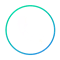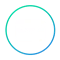• Overview
• Schedule Classes
• What you'll learn
• Curriculum
• Feature
• FAQsOverview

The Machine Learning Online Course gives the learner a holistic understanding of machine learning, covering theory, application, and inner workings of supervised, unsupervised, and deep learning algorithms. The course covers linear regression, K Nearest Neighbors, Clustering, SVM and neural networks using Python and R.

Schedule Classes

Looking for more sessions of this class?

What you'll learn

Upon completion of this course, a learner should be able to:
• Understand Machine Learning
• Carry out Data processing
• Perform Regression using Python and R
• Perform Classification using Python and R
• Clustering using Python and R, etc…
Duration: 32 Hours

Prerequisites

Basic Knowledge in R and Python is mandatory. It would be beneficial if the learner has Hadoop skills too

Curriculum

• What is Machine Learning?
• Applications of Machine Learning
• Why Machine Learning is the Future
• Installing R and R Studio (MAC & Windows)
• Installing Python and Anaconda (MAC & Windows)
• Data Preprocessing
• Importing the Libraries
• Importing the Dataset
• For Python learners, summary of Object-oriented programming: classes & objects
• Missing Data
• Categorical Data
• Splitting the Dataset into the Training set and Test set
• Feature Scaling
• Simple Linear Regression
• Dataset + Business Problem Description
• Simple Linear Regression in Python
• Simple Linear Regression in R
• Multiple Linear Regression
• Multiple Linear Regression in Python
• Multiple Linear Regression in R
• Polynomial Regression
• Polynomial Regression in Python
• Polynomial Regression in R
• Support Vector Regression (SVR)
• SVR in Python
• SVR in R
• Decision Tree Regression in Python
• Decision Tree Regression in R
• Random Forest Regression in Python
• Random Forest Regression in R
• Logistic Regression in Python and R
• K-Nearest Neighbors (K-NN)
• Support Vector Machine (SVM)
• Kernel SVM
• Naive Bayes
• Decision Tree Classification
• Random Forest Classification
• Confusion Matrix
• CAP Curve
• K-Means Clustering in Python and R
• Hierarchical Clustering in Python and R
• Association Rule Learning in Python and R
• Apriori
• Upper Confidence Bound (UCB)
• Thompson Sampling
• Natural Language Processing in R
• Natural Language Processing in Python
• Artificial Neural Networks in Python and R
• Convolution Neural Networks in Python and R

Interested in this course?+91-8511135819join@cognixia.com

Course FeatureCourse Duration24x7 SupportLifetime LMS AccessPrice match Guarantee

FAQs

Deep Learning has a wide horizon for IT professionals, electrical and electronics engineers, designers, and solution architects. It can also be a boon for the existing and budding entrepreneurs who are interested in building solutions for their customers. Professionals working in other sectors like pharmaceuticals, real estate, sales, finance, designing, manufacturing, electrical, retail, healthcare, etc. can also benefit from Machine Learning, AI & Deep Learning solutions. Graduates and newcomers can also kick-start their career with the Deep Learning
Yes, all our sessions are recorded. Therefore, if you ever miss a class, you will be able to view it on our LMS.
The course material is accessible for a lifetime, post-training
After you successfully complete the training program, you will be evaluated on parameters such as attendance in sessions, an objective examination, and other factors. Based on your overall performance, you will be certified by Cognixia.